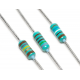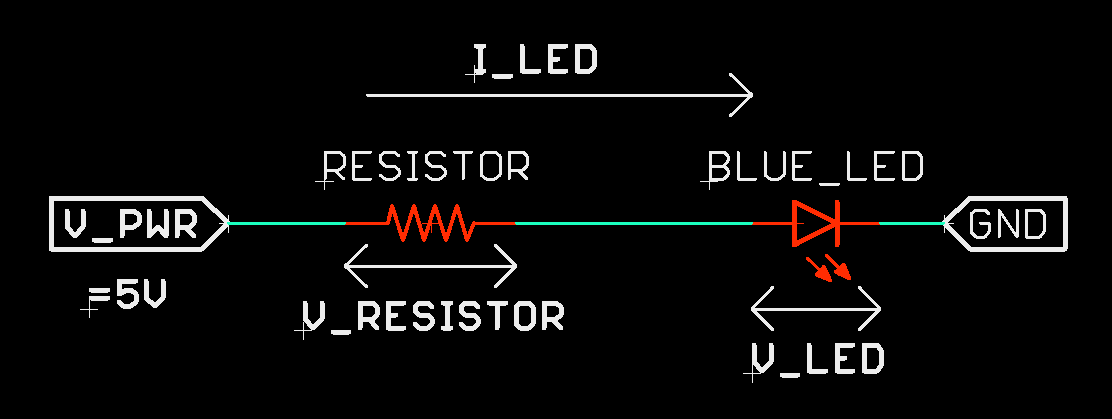## ResistorResistors act as the most common passive components in circuits.

For example, a resistor can be used to limit the current through a LED. In the example circuit given below, we can calculate the value of resistance by simple calculation as given below.

V_PWR-V_RESISTOR-V_LED=0

V_PWR-(I_LED*RESISTANCE VALUE)-V_LED =0

[Samples values => V_PWR=5V; I_LED=20mA; V_LED = 3V(typical forward voltage for blue LED)]

5-(0.02*RESISTANCE VALUE)-3=0

Which gives => RESISTANCE VALUE = 100 Ohm

In this case the power rating of the resistance also matter. Power rating of resistance is calculated below.

Power = (Square of Current) * (Resistance value)

Power = (0.02*0.02)*(100) = 0.04 Watt#### 1.5K-TH1/8W-5%

1.5K ohm through hole resistance in 1/8W package, with 5% tolerance...

Rs. 0.50

#### 100K-TH1/8W-5%

100 killo ohm through hole resistance in 1/8W package, with 5% tolerance...

Rs. 0.50

#### 100K_Trimpot

Resistance                         ..

Rs. 13.00

#### 100R-TH1/8W-5%

100 ohm through hole resistance in 1/8W package, with 5% tolerance...

Rs. 0.50

#### 10k-Potentiometer

A potentiometer is a three-terminal resistor with a sliding or rotating contact that forms..

Rs. 15.00

#### 10K-Preset

This adjustable or Variable resistor are PCB mountable and has 3 terminals. The voltage between the ..

Rs. 10.00

#### 10K-SMD0805-5%

10K Ohm SMD resistance in 0805 package, with 5% tolerance...

Rs. 1.00

#### 10K-TH1/4W-5%

10 kilo ohm through-hole resistance in 1/4W package, with 5% tolerance...

Rs. 0.55

#### 10K-TH1/8W-5%

10 killo ohm through hole resistance in 1/8W package, with 5% tolerance...

Rs. 0.50

#### 10K_Trimpot

Resistance                         ..

Rs. 13.00

#### 10M-TH1/8W-5%

10 Mega ohm through-hole resistance in 1/8W package, with 5% tolerance...

Rs. 0.50

#### 10R-TH1/2W-5%

10R TH Resistor through-hole resistance in 1/2W package, with 5% tolerance...

Rs. 1.20

#### 120K-TH1/8W-5%

120 killo ohm through hole resistance in 1/8W package, with 5% tolerance...

Rs. 0.50

#### 12R-SMD1206-5%

12 Ohm SMD resistance in 1206 package, with 5% tolerance...

Rs. 2.00

#### 150R-TH1/8W-5%

150 ohm through hole resistance in 1/8W package, with 5% tolerance...

Rs. 0.50

Showing 1 to 15 of 54 (4 Pages)